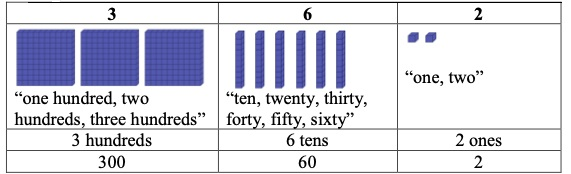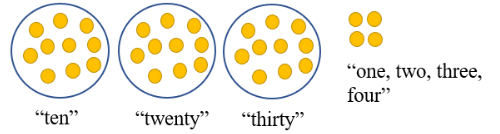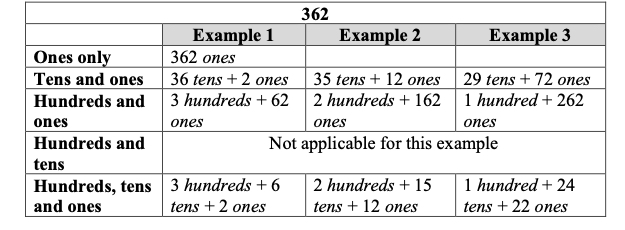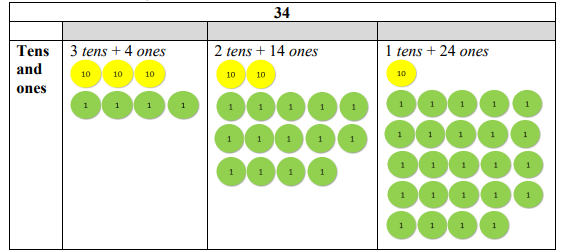# Standard #: MA.3.NSO.1.2

This document was generated on CPALMS - www.cpalms.org

Compose and decompose four-digit numbers in multiple ways using thousands, hundreds, tens and ones. Demonstrate each composition or decomposition using objects, drawings and expressions or equations.

### Examples

The number 5,783 can be expressed as 5 thousands + 7 hundreds + 8 tens + 3 ones or as 56 hundreds + 183 ones.

### General Information

Subject Area: Mathematics (B.E.S.T.)
Strand: Number Sense and Operations
Status: State Board Approved

### Terms from the K-12 Glossary

• Expression
• Whole numbers

### Vertical Alignment

Previous Benchmarks

Next Benchmarks

### Purpose and Instructional Strategies

The purpose of this benchmark is for students to identify ways numbers can be written flexibly using decomposition. In addition to students knowing that number sense and computational understanding is built on a firm understanding of place value. This work extends from the Grade 2 expectation to compose and decompose three-digit numbers in multiple ways using hundreds, tens and ones (MA.2.NSO.1.2).
• Multiple representations of multi-digit whole numbers allow students to identify opportunities for regrouping while adding and subtracting. For example, when subtracting 5,783 – 892, we can represent 5,783 as 5 thousands + 6 hundreds + 18 tens + 3 ones by regrouping 1 hundred as 10 tens, allowing us to subtract 9 tens (MTR.2.1, MTR.3.1).
• Students should use objects (e.g., base ten blocks), drawings, and expressions or equations side-by-side to see compare and contrast the representations. Model to show how multiple representations relate to the original number. For example, use base ten blocks to show how in the number 5,783, 1 hundred can be regrouped as 10 tens to express it as 5 thousands + 6 hundreds + 18 tens + 3 ones, while asking students how they are the same (MTR.2.1).
• Allow students to decompose numbers in as many ways as possible. Have students compare and contrast the representations shared (MTR.4.1).
• Students should see examples of numbers within 10,000 where zero is a digit and make sense of its value.
• Flexibility of place value is a prerequisite for conceptual understanding of a standard algorithm for addition and subtraction with regrouping.

### Common Misconceptions or Errors

• Students can misunderstand that the 5 in 57 represents 5, not 50 or 5 tens. Students need practice with representing two and three-digit numbers with manipulatives that group (base ten blocks) and those that do NOT group, such as counters, etc.
• Students can misunderstand that when decomposing a number in multiple ways, the value of the number does not change. 879 is the same as 87 tens + 9 ones and 8 hundreds + 79 ones.

### Strategies to Support Tiered Instruction

• Instruction includes decomposing numbers using manipulatives that group (base ten blocks) and those that do not group such as counters. When decomposing a number, students focus on the value of each digit based on its place value. To reinforce this concept, students may count by units based on the place value.
• For example, decompose 362 using base ten blocks and explain the value of each digit.• For example, represent 34 using counters and explain the value of each digit. Students group 10 ones as a group of ten and focus on the value of each digit based on its place value. To reinforce this concept, students count by units based on the place value.• Teacher provides opportunities to decompose numbers in multiple ways using manipulatives and a chart to organize their thinking and asks students to name/identify the different ways to name the values (regrouping the hundreds into tens and the tens into the ones, e.g., 36 tens and 2 ones or 3 hundreds and 62 ones, etc.)
• For example,  students decompose 362 in multiple ways using hundreds, tens, and ones.• For example, students decompose 34 in multiple ways using tens and ones.• Express the number 5,783 using only hundreds and ones.

• Express the number 5,783 using only thousands and hundreds.

• Express the number 5,783 using only tens and ones.

### Instructional Items

Instructional Item 1

• Select all the ways that express the number 8,709.
• a. 8,000 + 600 + 19
• b. 8,000 + 700 + 9
• c. 879 ones
• d. 8 thousands + 6 hundreds + 10 tens + 9 ones
• e. 8 thousands + 7 tens + 9 ones
*The strategies, tasks and items included in the B1G-M are examples and should not be considered comprehensive.

#### Related Courses

 Course Number1111 Course Title222 5012050: Grade Three Mathematics (Specifically in versions: 2014 - 2015, 2015 - 2022, 2022 and beyond (current)) 7712040: Access Mathematics Grade 3 (Specifically in versions: 2014 - 2015, 2015 - 2018, 2018 - 2022, 2022 and beyond (current)) 5012055: Grade 3 Accelerated Mathematics (Specifically in versions: 2019 - 2022, 2022 and beyond (current)) 5012015: Foundational Skills in Mathematics 3-5 (Specifically in versions: 2019 - 2022, 2022 and beyond (current))

#### Related Access Points

 Access Point Number Access Point Title MA.3.NSO.1.AP.2 Compose and decompose three-digit numbers using hundreds, tens and ones. Demonstrate each composition or decomposition with objects, drawings, expressions or equations.

#### Lesson Plans

 Name Description What Decade is It? - Rounding Whole Numbers to the Nearest 10 In this lesson, students will tap prior knowledge of place value and dimes to round numbers to the nearest 10. Students will use number lines with tick marks in increments of 10 to visualize the closer decade and then progress to using the place value of numerals to round to the tens place. Pairs of students will participate in a rounding activity using a number line. Jumping Beans: Adding with Open Number Lines Students will use the open number line as a strategy to add within 1,000. The lesson begins with a formative assessment that evaluates students' strategies for efficiently solving addition sentences within 1,000. In order for students to have practice reinforcing the skill, students will complete the guided practice with open number lines, as well as playing the game, Jumping Beans. To conclude the lesson, students will evaluate a solution to an open number line problem. Decoding Decomposing {Adding two 3-digit Numbers} Students will add 3-digit numbers by decomposing them by place value.  It is a useful lesson to reinforce place value concepts when adding.

#### Tutorial

 Name Description Regrouping Numbers: 4,500 = 3 thousands + ? hundreds In this tutorial, you will look at regrouping a number by different place values.

#### Tutorial

 Name Description Regrouping Numbers: 4,500 = 3 thousands + ? hundreds: In this tutorial, you will look at regrouping a number by different place values.

Printed On:12/5/2023 6:36:17 PM
Print Page | Close this window